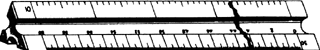engineer's scale

Also found in: Wikipedia.

engineer's scale

[‚en·jə′nirz ‚skāl]
(graphic arts)
A rule having a triangular cross section and different measurement scales on the two edges of each face.

engineer’s scaleengineer’s scale
A straightedge, divided uniformly into multiples of 10 divisions per inch so that drawings may be made with decimal values of distances, loads, forces, etc.
Mentioned in ?
References in periodicals archive ?
Distances can be readily scaled by using the 60 scale on the Engineer's scale. Having photographs taken periodically in this uniform scale makes plotting of construction progress on the map very easy and reduces materially the extensive field surveys normally used for this purpose.
Gridded vellum contains a grid of light blue lines that will not reproduce at regular photocopy or diazo (blueprinting) process contrast settings and is available for different scales (8 lines per inch for architect's scale drawings and 10 lines per inch for engineer's scale drawings).
Two scales that designers commonly use are the architect's scale and the engineer's scale (Figure 1-15; Figure 1-16).
The engineer's scale is divided into decimal parts, or units of 10.
Two types of scales are used for construction drawings--an architect's scale using fractional divisions, and an engineer's scale using multiples of ten (Figure 3-1).
Measuring lines with engineer's scales also requires determining drawing scale before measurement.
Two types of scales are commonly used by landscape designers: the engineer's scale and the architect's scale.
Depending upon the desired size of the drawing or the size of the property that must be represented on paper, a scale will be selected for the drawing to correspond with one of the six edges on either the architect's or engineer's scale instrument.
Line Length to Represent 100 feet Scale of Architect's Engineer's the Plan Scale Scale 1" = 10' 10 inches 1" = 20' 5 inches 1" = 40' 2-1/2 inches 1/16" = 1' 6-1/4 inches 1/8" = 1' 12-1/2 inches 1/4" = 1' 25 inches In Figure 1-17, the same measurement of 60 feet is located on each of the six sides of an engineer's scale. Note that each unit represents 1 linear foot regardless of the side of the instrument being used, as long as the scale of the drawing matches the side of the scale instrument.
Two types of scales are typically used for plans: an architect's scale using fractional divisions, and an engineer's scale, using multiples of ten.
Large projects like an entire yard could be drawn in engineer's scale. Engineer's scales use 1" (on the plan) = 10' (on the actual site, 1" = 20', and similar increments, up to 1" = 60'.
We use an engineer's scale to make measurements on the map, and we expect that our measurements are accurate to within a foot over the 480-ft length, represented by the 12-in.

Site: Follow: Share:
Open / Close